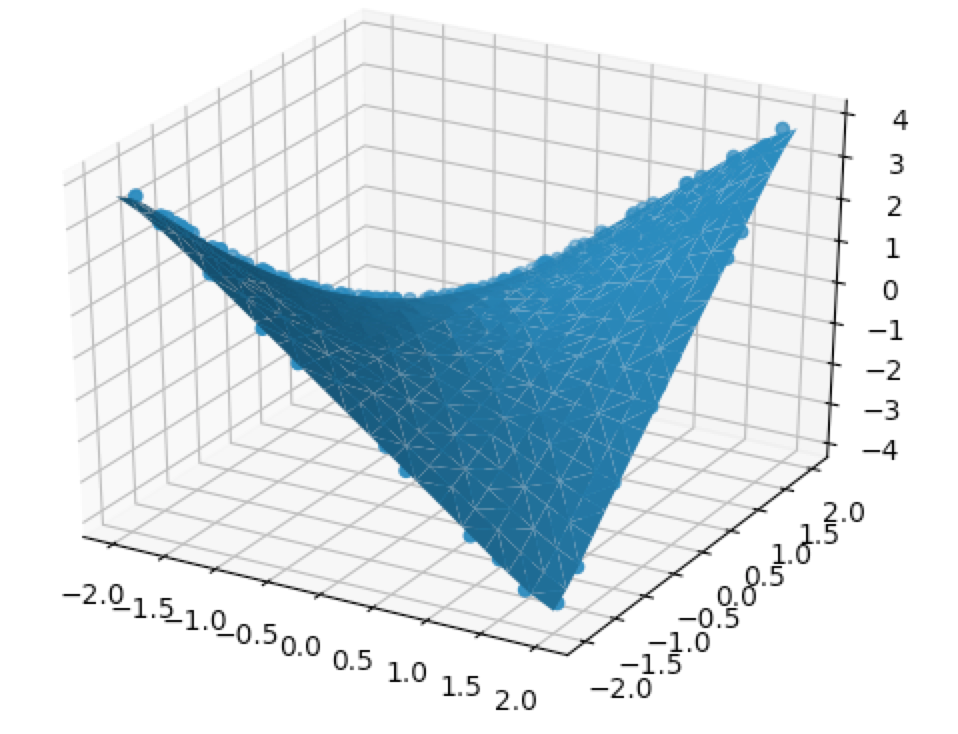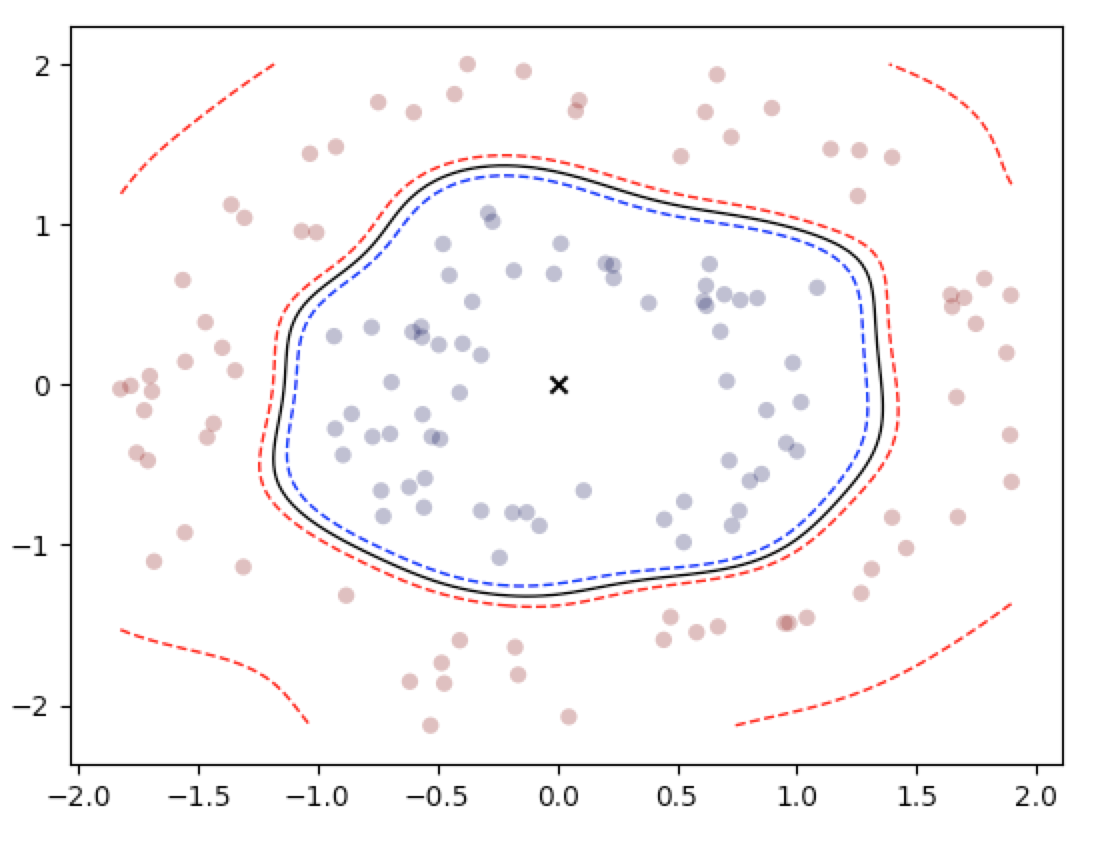# Machine Learning and AI: Support Vector Machines in Python

### Course Data

Lectures: 73
Length: 8h 53m
Skill Level: All Levels
Languages: English

### Course Description

Support Vector Machines (SVM) are one of the most powerful machine learning models around, and this topic has been one that students have requested ever since I started making courses.

These days, everyone seems to be talking about deep learning, but in fact there was a time when support vector machines were seen as superior to neural networks. One of the things you’ll learn about in this course is that a support vector machine actually is a neural network, and they essentially look identical if you were to draw a diagram.

The toughest obstacle to overcome when you’re learning about support vector machines is that they are very theoretical. This theory very easily scares a lot of people away, and it might feel like learning about support vector machines is beyond your ability. Not so!

In this course, we take a very methodical, step-by-step approach to build up all the theory you need to understand how the SVM really works. We are going to use Logistic Regression as our starting point, which is one of the very first things you learn about as a student of machine learning. So if you want to understand this course, just have a good intuition about Logistic Regression, and by extension have a good understanding of the geometry of lines, planes, and hyperplanes.

This course will cover the critical theory behind SVMs:

• Linear SVM derivation
• Hinge loss (and its relation to the Cross-Entropy loss)
• Quadratic programming (and Linear programming review)
• Slack variables
• Lagrangian Duality
• Kernel SVM (nonlinear SVM)
• Polynomial Kernels, Gaussian Kernels, Sigmoid Kernels, and String Kernels
• Learn how to achieve an infinite-dimensional feature expansion
• SMO (Sequential Minimal Optimization)
• RBF Networks (Radial Basis Function Neural Networks)
• Support Vector Regression (SVR)
• Multiclass Classification

As a bonus, you will also get material for how to apply the "Kernel Trick" to other machine learning models. This is how you can use a model which is normally "weak" (such as linear regression) and make it "strong". I've chosen models from various different areas of machine learning.

• Kernel Linear regression (for regression)
• Kernel Logistic regression (for classification)
• Kernel K-means clustering (for clustering)
• Kernel Principal components analysis (PCA) (for dimensionality reduction)

See here what linear regression can be capable of:And logistic regression:When the kernel trick is applied!

For those of you who are thinking, "theory is not for me", there’s lots of material in this course for you too!

In this course, there will be not just one, but two full sections devoted to just the practical aspects of how to make effective use of the SVM.

We’ll do end-to-end examples of real, practical machine learning applications, such as:

• Image recognition
• Spam detection
• Medical diagnosis
• Regression analysis

For more advanced students, there are also plenty of coding exercises where you will get to try different approaches to implementing SVMs.

These are implementations that you won't find anywhere else in any other course.

Thanks for reading, and I’ll see you in class!

Suggested Prerequisites:

• calculus
• probability
• Python coding: if/else, loops, lists, dicts, sets